Click to Chat

1800-1023-196

+91-120-4616500

CART 0

• 0

MY CART (5)

Use Coupon: CART20 and get 20% off on all online Study Material

ITEM
DETAILS
MRP
DISCOUNT
FINAL PRICE
Total Price: Rs.

There are no items in this cart.
Continue Shopping• Complete Physics Course - Class 11
• OFFERED PRICE: Rs. 2,968
• View Details

Conservation of Linear Momentum

Table of Content

Alternative Method

Applications of conservation of momentum

Recoil of gun

Rocket and jet plane

Explosion of a bomb

A man Jumping from a boat

Some Conceptual Questions

Some Solved Problems

Related Resources

It states that,

“In an isolated system (no external force), the algebraic some of the momenta of bodies, along any straight line, remains constant and is not changed due to their mutual action and reaction on each other”.

This can be verified by a following simple experiment.

Consider a body ‘A’ of mass ‘m1’ moving with a velocity   strike against another body ‘B’ of mass m2,moving with velocity  in same direction as shown in the below figure. Two bodies remain in contact with each other for small time ‘?t’. They get separated and move with velocities  and  after collision.Let  be the force exerted by ‘A’ upon ‘B’ and be its reaction. Since the system is isolated, i.e., no external force is there,

So,

…... (1)

This is in accordance with Newton’s third law of motion that ‘action and reaction are equal and opposite’.

Considering the momenta of the bodies before and after collision.

Body A

Body B

Momentum of A before collision =

Momentum of A after collision =

Change in momentum of A =

Time taken for the change of momentum =?t

Rate of change of momentum of A (=Force on A) =

So,

Momentum of A before collision =

Momentum of B after collision =

Change in momentum of B =

Time taken for the change of momentum =?t

Rate of change of momentum of B (=Force on B)=

So,

Substituting for  and  in equation (1),

Or,

Thus, the total momentum of the system before collision is equal to the total momentum of the system after collision.

This verifies the law of conservation of momentum.

It may be noted that the conservation of momentum is closely connected with the validity of Newton’s third law of motion, since we have used equation (1) [which is nothing but third law] to prove it.

Alternative Method

According to Newton’s second law of motion,

Since  (momentum of body),

Incase of an isolated system,

Thus,

or,

Therefore, momentum (in vector form) of an isolated system remains constant. This is in accordance with the law of conservation of momentum.

IMPORTANT NOTE:-

While applying law of conservation of momentum to a system following consideration must be kept in mind:

(a) The system must be isolated.

(b) While finding the algebraic sum of momenta it must be ensured that all of them are along a particular straight line.

Applications of Conservation of Momentum

Following few examples with illustrate the law of conservation of momentum.

(a) Recoil of GunA gun and a bullet constitute one isolated system. On firing the gun, bullet moves out with a very high velocity . The gun experiences a recoil. It moves in the opposite direction as shown in the below figure. Velocity ‘’ of the recoil gun can be calculated by the application of law of conservation of momentum.

Before Firing

After Firing

Momentum of bullet = 0

Momentum of gun = 0

Total momentum of the system = 0

Momentum of bullet =

Momentum of gun =

Total momentum of the system =

Here ‘m’ and ‘M’ are the masses of bullet and gun respectively. According to the law of conservation of momentum, momentum before collision and after collision must be same.

or,

or,

Negative sign indicates that direction of motion of gun is in opposite direction.

(b) Rocket and Jet Plane

Fuel and oxygen is burnt in the ignition chamber. As hot gases escape from a rear opening, with some momentum, the rocket moves in the forward direction with the same momentum.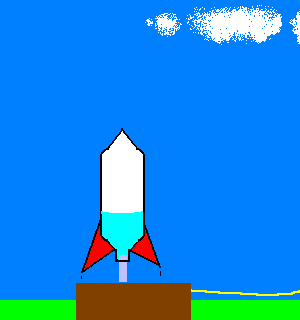(c)  Explosion of a Bomb

Momentum of a bomb before explosion is zero. After explosion different fragments fly in various directions. It will be observed that their momenta, when represented by the slide of a polygon, from a closed polygon, indicating that net momentum after explosion is also zero. Thus, if the bomb exploded into two fragments, they must move in opposite directions.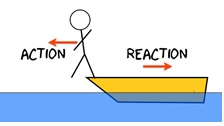(d) A man Jumping from a Boat

When a man jumps from the boat to the shore, the boat is pushed backward. It can, exactly, be explained as in the case of recoil of gun.

Some Conceptual Questions

Question 1:-

Figure below shows a popular carnival device, in which the contestant tries to see how high a weighted marker can be raised by hitting a target with a sledge hammer. What physical quantity does the device measure? Is it the average force, the maximum force, the work done, the impulse, the energy transferred, the momentum transferred, or something else? Discuss your answer.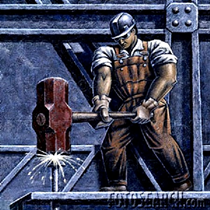The device will measure impulse. The impulse of the net force acting on a particle during a given time interval is equal to the change in momentum of the particle during that interval. Since the contestant is hitting the target with a sledge hammer the change in momentum is large and the time of collision is small, therefore it signifies that the average impulsive force will relatively large. Suppose two persons bring the harmer from the same height, but they are hitting with different forces. The person who hits with greater force for the short time interval the impulse will be more and this results the height of the mark will be more. Thus the device will measure impulse.

________________________________________________________________________________________________________________

Question 2:-

Can the impulse of a force be zero, even if the force is not zero? Explain why or why not?

Yes, the impulse of a force can be zero, even if the force is not zero.

Impulse of a force is defined as the change in momentum produced by the force and it is equal to the product of force and the time for which it acts. The impulse of a force can be zero, if the net force acting on the particle during that time interval is constant. Since the force is constant (both magnitude and direction), so change in momentum produced by the force will be zero. Therefore impulse of the force will be zero.

From the above observation we conclude that, impulse of a force can be zero, even if the force is not zero.

__________________________________________________________________________________________________________________

Question 3:-

Explain how conservation of momentum applies to a handball bouncing off a wall.

Law of conservation of linear momentum states that, in an isolated system (no external force), the algebraic sum of momenta of bodies, along any straight line, remains constant and is not changed due to their mutual action and reaction on each other.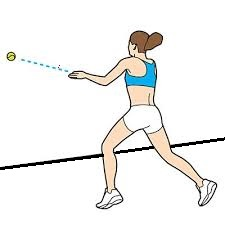The momentum of particle (p) is equal to the mass of particle (m) times the velocity of particle (v).

So,p = mv    …… (1)

Let us consider m is the mass of the ball and v is the velocity of the ball when the ball is collides with wall.

So using equation (1), the momentum of the ball before collision (p1) will be,

p1= mv          …… (2)

After collision, when the ball re bounces, the velocity of the ball will be, -v.

So again using equation (1), the momentum of the ball after collision (p2) will be,

p2= -mv            …… (3)

Conservation of linear momentum states that, the algebraic sum of momenta of bodies, along any straight line, remains constant and is not changed due to their mutual action and reaction on each other.

p1 + p2 = 0

So, mv + (-mv) = 0        …… (4)

From equation (4) we observed that, linear momentum of the hand ball is conserved.

_________________________________________________________________________________________________________

Question 4:-

Give a plausible explanation for the breaking of wooden boards or bricks by a karate punch. (See “Karate Strikes.” by Jearl D. Walker, American Journal of Physics, October 1975, p.845.)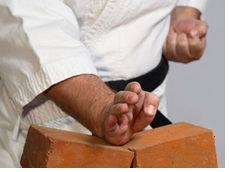In the process, breaking of wooden boards or bricks by a karate punch, the collision between the hand and brick is only for a few milliseconds. Because the applied external force is large and the time of collision is small therefore the average impulsive force is relatively large. Thus when you break a wooden board or bricks by a karate punch you have to apply large force for the minimum time which is impulse. Therefore the impact force on the brick or wooden boards will be high.

Some Solved Problems

Problem 1:-

A 75.2-kg man is riding on a 38.6-kg cart travelling at a speed of 2.33 m/s. He jumps off in such a way as to land on the ground with zero horizontal speed. Find the resulting change in the speed of the cart.

Concept:-

Momentum of the body p is equal to the mass of the body m times velocity of the body v.

So, p = mv

In accordance to the principle of conservation of energy, the final momentum of the system is equal to the initial momentum of the system.

Consider the initial momentum of the man is pi,m, initial momentum of the cart is pi,c, final momentum of the man is pf,m and final momentum of the cart is pf,c.

We define,  pf,m = mmvf,m

pf,c = mcvf,c

pi,m = mmvi,m

pi,c = mcvi,c

Here, mass of the man is mm, mass of the cart is mc, initial velocity of the man is vi,m and cart is vi,c, and final velocity of the man is vf,m and cart is vf,c.

Solution:-

So applying conservation of momentum to this system, the sum of the initial momentum of the man and cart will be equal to the sum of the final momentum of the man and cart.

pf,m + pf,c = pi,m + pi,c

Substitute, mmvf,m for pf,m, mcvf,c for pf,c, mmvi,m for pi,m and mcvi,c for pi,c ijn the equation pf,m + pf,c = pi,m + pi,c,

pf,m + pf,c = pi,m + pi,c

mmvf,m + mcvf,c = mmvi,m + mcvi,c

vf,c- vi,c = (mmvi,m - mmvf,m)/ mc

Δvc = (mmvi,m - mmvf,m)/ mc

To obtain the resulting change in the speed of the cart Δvc, substitute 75.2 kg for mm, 2.33 m/s for vi,m and 0 m/s for vf,m in the equation Δ vc = (mmvi,m - mmvf,m)/ mc,

Δvc = (mmvi,m - mmvf,m)/ mc

= (75.2 kg) (2.33 m/s) – (75.2 kg) (0 m/s)/(38.6 kg)

= 4.54 m/s

As the sign of the change in the speed of the cart Δvc is positive, this signifies that, the cart speed increases.

From the above observation we conclude that, the resulting change in the speed of the cart Δvc would be 4.54 m/s.

___________________________________________________________________________________________________________

Problem 2:-

A space vehicle is travelling at 3860 km/h with respect to the Earth when the exhausted rocket motor is disengaged and sent backward with a speed of 125 km/h with respect to the command module. The mass of the motor is four times the mass of the module. What is the speed of the command module after the separation?

Concept:-

Momentum of the body p is equal to the mass of the body m times velocity of the body v.

So, p = mv

In accordance to the principle of conservation of energy, the final momentum of the system is equal to the initial momentum of the system.

Consider the initial momentum of the motor is pi,m, initial momentum of the command module is pi,c, final momentum of the motor is pf,m and final momentum of the command module is pf,c.

Initial momentum of the motor is equal to the initial momentum of the command module.

So, initial momentum of the motor will be,

pi,m = mmvi,c

and final momentum of the motor pf,m will be,

pf,m = mm(vf,c – vrel)

Here, speed of the motor relative to the command module is vrel.

Again, pf,c = mcvf,c

pi,c = mcvi,c

Here, mass of the motor is mm, mass of the command module is mc, initial velocity of the motor is vi,m and command module is vi,c, and final velocity of the motor is vf,m and command module is vf,c.

Solution:-

So applying conservation of momentum to this system, the sum of the initial momentum of the motor and command module will be equal to the sum of the final momentum of the motor and command module.

pf,m + pf,c = pi,m + pi,c

Substitute, mm(vf,c – vrel) for pf,m, mcvf,c for pf,c, mmvi,c for pi,m and mcvi,c for pi,c in the equation pf,m + pf,c = pi,m + pi,c,

pf,m + pf,c = pi,m + pi,c

mm(vf,c – vrel)+ mcvf,c = mmvi,c + mcvi,c

(mm + mc)vf,c - mm vrel = (mm +mc) vi,c

(mm + mc)vf,c = mm vrel + (mm +mc) vi,c

So, vf,c = [mm vrel + (mm +mc) vi,c]/ (mm + mc)

As, mass of the motor is four times the mass of the module, thus,

mm = 4 mc

To obtain the speed of the command module vf,c, substitute 4 mc for mm, 125 km/h for vrel and 3860 km/h for vi,c in the equation vf,c = [mm vrel + (mm +mc) vi,c]/ (mm + mc),

vf,c = [mm vrel + (mm +mc) vi,c]/ (mm + mc)

= [4 mc(125 km/h) + (4 mc+mc) (3860 km/h)]/ [4 mc+ mc]

= 3960 km/h

From the above observation we conclude that, the speed of the command module vf,c would be 3960 km/h.

Related Resources

You might like to refer Momentum.

For getting an idea of the type of questions asked, refer the Sample Papers of Previous Years.### Course Features

• 101 Video Lectures
• Revision Notes
• Previous Year Papers
• Mind Map
• Study Planner
• NCERT Solutions
• Discussion Forum
• Test paper with Video Solution